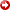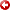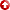[First Hit]# Carbonate Reservoir Characterization UsingElectricalBorehole Image – Revisited ExistingMethodsUsing Textural Analysis Workflow

## Abstract

Carbonate formations provide the main reservoirs in the Middle East Region. Many productive carbonate formations have complex dual porosity system consisting of primary matrix porosity and secondary porosity. The secondary porosity may comprise vugs, molds and fractures.Electricalborehole images provide both high resolution and azimuthal coverage to quantify the heterogeneous nature of the carbonate porosity. Porosity Spectrum Analysis (PoroSpect) is a textural analysis technique that gives porosity distribution and vug fraction quantification from high-resolutionelectricalborehole images such as the Formation Micro-Imager (FMI). The primary assumption for this technique is that the conductivity measurement from theelectricalimages is measured in the flushed zone of the borehole. After calibration with a shallow resistivity log, the conductivity images are transformed into a porosity map of the borehole using log porosity. The following equation is used to get such transformation (Akbar et al., 2000). Φ i = Φlog [RLLS * Ci] 1/m (Where Ci is conductivity of each button from the image, RLLS is external shallow flushed zone resistivity curve, Φlog is external porosity curve, m is Archie cementation exponent and Φi is derived porosity for each button of the image). A histogram of porosities over a short depth window (typically 1.2”) is computed. Differentmethodsare then used to compute a threshold between the primary and secondary porosity. (1) In the Newberry method (Newberry et al., 1996), the standard deviation of the porosity distribution is computed and a threshold is obtained by adding a multiple of this standard deviation to the median porosity. (2) The SDR or Fixed-Percentage method locates the threshold at a fixed percentage above the mean porosity. (3) The TSR Discriminant method (Ramakrishna et al., 2001) does not require any user input to define the threshold. It involves decomposing a distribution using linear discriminant analysis (LDA). This method works by grouping the data into classes by minimizing within-class scatter and maximizing between-class scatter so that within a group data are “similar” while between groups they are “different”. Simply stated, if the porosity data consists of two populations, then the best threshold should maximally separate the two means. (4) The Gaussian Extraction Optimization-based method (Goswami et al., 2004) involves multimodal decomposition of a composite distribution, in this case applied to the porosity distribution. The porosity distribution can considered as a superposition of several distributions, each corresponding to some type of pore configuration. The method selects one of the identified Gaussian distributions, classifies it as secondary porosity and computes secondary porosity by integrating the distribution. (5) The manual method which allows user to manually set a fixed threshold per zone. The best threshold should separate the primary and secondary porosity.